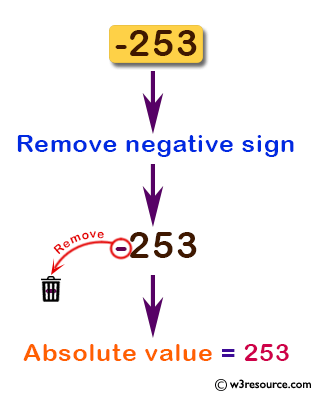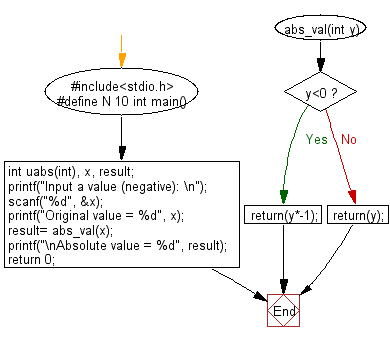﻿ C Program: Remove any negative sign in front of a number - w3resource

# C Exercises: Remove any negative sign in front of a number

## C Basic Declarations and Expressions: Exercise-72 with Solution

Write a C program to remove any negative sign in front of a number.

Pictorial Presentation:Sample Solution:

C Code:

``````#include<stdio.h>
#define N 10
// Function prototype declaration
int abs_val(int);

int main() {
int x, result;

// Prompt for user input
printf("Input a value (negative): \n");
scanf("%d", &x);
printf("Original value = %d", x);

// Call the abs_val function and store the result
result = abs_val(x);

// Output the result
printf("\nAbsolute value = %d", result);

return 0;
}

// Function to calculate the absolute value
int abs_val(int y) {
if(y < 0)
return(y * -1);
else
return(y);
}
``````

Sample Output:

```Input a value (negative):
Original value = -253
Absolute value = 253
```

Flowchart:C programming Code Editor:

What is the difficulty level of this exercise?

Test your Programming skills with w3resource's quiz.

﻿